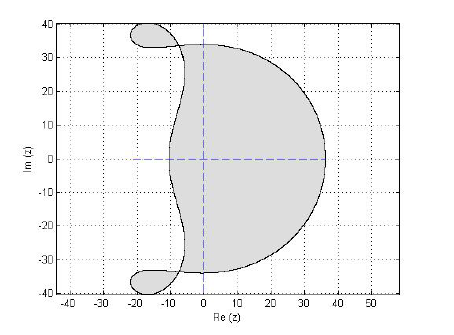# A Class of Block Multi-derivative Numerical Techniques for Singular Advection Equations

• M. O. Ogunniran Department of Mathematical Sciences, Osun State University Osogbo, Nigeria
Keywords: Advection equations, Singular point, Multi-derivative, Conservation law, off-step point

### Abstract

The integration of some differential equations is hard to acquire because of the presence of singular point(s) in these equations. These equations are best solved by some unique technique. Multi-derivative techniques have a long history of powerful integration of such equations yet till date, a couple of class of this technique has been explored for integrating partial differential equations. This work centers around the development, analysis, and implementation of a class of multi-derivative technique on partial differential equations. The approaches were effectively analyzed and were turned out to be consistent, stable and convergent. Numerical outcomes got likewise demonstrated the approximation quality of the technique over existing techniques in the literature.

### References

A. Balzano, “Mosquito: An Efficient Finite Difference Scheme for Numerical Simulation of 2D Advection Equation”, International Journal for Numerical Methods in Fluids 32 (1999) 2.

B. J. Noye and M. Dehghan, ”New explicit finite difference schemes for two-dimensional diffusion subject to specification of mass”, Numerical Methods For Partial Differential Equations 15 (1999) 4.

Y. Han, X. Zhang, L. Liu, Y. and Wu, ”Multiple positive solutions of singular nonlinear Sturm-Liouville problems with Caratheodory perturbed term”, Journal of Applied Mathematics, (2012) 160891.

F. Z. Geng and S. P. Qian, ”Solving singularly perturbed multipantograph delay equations based on the reproducing kernel method”, Abstract and Applied Analysis, (2014) 794716.

M. Stojanovic, ”Splines difference methods for a singular perturbation problem”, Applied Numerical Mathematics 21 (1996) 3.

S. Bushnaq, B. Maayah, S. Momani, and A. Alsaedi, “A Reproducing Kernel Hilbert Space Method for Solving Systems of Fractional Integro differential Equations”, Abstract and Applied Analysis (2014) 103016.

Z. Xianyl, ”Linear Hybrid-variable Methods for Advection Equations”, Advances in Computational Mathematics, Journal of Differential Equations, Conf 23 (2016) https://doi.org/10.1007/s10444-018-9647-z.

E. Utku, S. Murat and K. Huseyin, “Efficient Numerical Treatment of Nonlinearities in the Advection-Diffusion-Reaction Equation”, International Journal of Numerical Methods for Heat and Fluid Flow, http://doi.org/10.1108/HFF-02-2017-0198.

Z. Gaetamo and P. Silvia, “On the Fractional Diffusion-Advection Equation for Fluids and Plasmas”, Fluid 4 (2019) 62.

S. Adrian, “A Generalization of Prefactored Compact Schemes for the Solution of Adevection Equations”, arXiv:1902.04429v1 math. NA (2019).

Y. Wang, H. Yu, G. Tan, and S. Qu, “Solving a Class of Singularly Perturbed Partial Differential Equations by Using the Perturbation Method and Reproducing Kernel Method”, Hindawi Abstract and Applied Analysis (2014) 5.Published
2019-10-25
How to Cite
Ogunniran, M. O. (2019). A Class of Block Multi-derivative Numerical Techniques for Singular Advection Equations. Journal of the Nigerian Society of Physical Sciences, 1(2), 62-71. Retrieved from https://journal.nsps.org.ng/index.php/jnsps/article/view/12
Section
Original Research